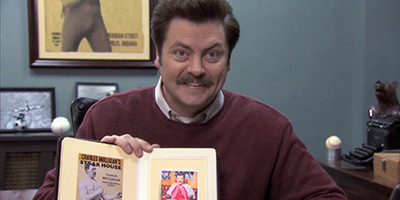## Exercise 1

Before it closed, Ron Swanson was a frequent patron of Charles Mulligan’s Steakhouse in Indianapolis, Indiana. Ron enjoyed the experience so much, during each visit he took a picture with his steak.Ron also weighed each steak he consumed. He has a record of eating six “22 ounce” Charles Mulligan’s porterhouse steaks. Ron found that these six steaks weighed

$22.4 \text{ oz}, \ 20.8 \text{ oz}, \ 21.6 \text{ oz}, \ 20.2 \text{ oz}, \ 21.4 \text{ oz}, \ 22.0 \text{ oz}$

Suppose that “22 ounce” Charles Mulligan’s porterhouse steaks follow a $$N(\mu, \sigma^2)$$ distribution and that Ron’s six steaks were a random sample.

Recall that

$\bar{x} = 21.4 \quad \text{and} \quad s^2 = 0.64.$ (a) Calculate the test statistic for testing $$H_0: \ \mu = 22$$, where $$\mu$$ is the true weight of a “22 ounce” Charles Mulligan’s porterhouse. Report your answer rounded to three decimal places.

(b) Calculate the p-value for the test $$H_0: \ \mu = 22$$ versus $$H_1: \ \mu < 22$$. Report your decision when $$\alpha = 0.05$$.

Some useful code:

• Excel: T.DIST(x, df, 1) gives the area to the left of x.
• Excel: TDIST(x, df, 1) gives the area to the right of x. (An older command. Only accepts positive values for x.)
• R: pt(x, df) gives the area to the left of x.

(c) Calculate the test statistic for testing $$H_0: \ \sigma \leq 0.50$$, where $$\sigma$$ is the true standard deviation of the weight of a “22 ounce” Charles Mulligan’s porterhouse.

(d) Calculate the p-value for the test $$H_0: \ \sigma \leq 0.50$$ versus $$H_1: \ \sigma > 0.50$$. Report your decision when $$\alpha = 0.01$$.

Some useful code:

• Excel: CHISQ.DIST(x, df, 1) gives the area to the left of x.
• Excel: CHIDIST(x, df, 1) gives the area to the right of x. (An older command.)
• R: pchisq(x, df) gives the area to the left of x.

(e) Suppose the true value of $$\sigma$$ is indeed 0.50. Was your decision in part (d) correct? If not, what Type of error was made? Justify your answer.

## Exercise 2

Last year, ballots in Champaign-Urbana contained the following question to assess public opinion on an issue:

“Should the State of Illinois legalize and regulate the sale and use of marijuana in a similar fashion as the State of Colorado?”

Suppose that we would like to understand Champaign-Urbana’s 2017 opinion on marijuana legalization. To satisfy our curiosity, we obtain a random sample of 120 Champaign-Urbanians and find that 87 support marijuana legalization.

(a) Calculate the $$z$$ test statistic for the test $$H_0: \ p = 0.70$$ versus $$H_1: \ p > 0.70$$ where $$p$$ is the true proportion of Champaign-Urbanians that support marijuana legalization. Report your answer rounded to two decimal places.

(b) Calculate the (approximate, using $$z$$) p-value for the test $$H_0: \ p = 0.70$$ versus $$H_1: \ p > 0.70$$.

(c) Calculate the exact p-value for the test $$H_0: \ p = 0.70$$ versus $$H_1: \ p > 0.70$$. That is, calculate the probability of seeing as many supporters as observed, or more, assuming the null hypothesis is true.

Some useful code:

• Excel: BINOM.DIST(x, n, p, 1) gives the probability of less than or equal to x.
• R: pbinom(x, n, p) gives the probability of less than or equal to x.

# Exercise 3

Last year, ballots in Champaign-Urbana contained the following question to assess public opinion on an issue:

“Should the State of Illinois legalize and regulate the sale and use of marijuana in a similar fashion as the State of Colorado?”

Suppose we obtain a random sample of 80 Champaign voters, of which 55 support marijuana legalization. We also obtain a random sample of 100 Urbana voters, of which 75 support marijuana legalization. Let $$p_C$$ be the true proportion of Champaign voters who support marijuana legalization and let $$p_U$$ be the true proportion of Urbana voters who support marijuana legalization.

(a) Calculate a 99% confidence interval for $$p_U - p_C$$.

(b) Calculate the p-value for the test $$H_0: \ p_U = p_C$$ versus $$H_1: \ p_U \neq p_C$$.